# Hyperparameter Tuning of Decision Tree Classifier Using GridSearchCV

## The models can have many hyperparameters and finding the best combination of the parameter using grid search methods.

### What is Grid Search?

Grid search is a technique for tuning hyperparameter that may facilitate build a model and evaluate a model for every combination of algorithms parameters per grid. We might use 10 fold cross-validation to search the best value for that tuning hyperparameter. Parameters like in decision criterion, max_depth, min_sample_split, etc. These values are called hyperparameters. To get the simplest set of hyperparameters we will use the Grid Search method. In the Grid Search, all the mixtures of hyperparameters combinations will pass through one by one into the model and check the score on each model. It gives us the set of hyperparameters which gives the best score. Scikit-learn package as a means of automatically iterating over these hyperparameters using cross-validation. This method is called Grid Search.

### How does it work?

Grid Search takes the model or objects you'd prefer to train and different values of the hyperparameters. It then calculates the error for various hyperparameter values, permitting you to choose the best values.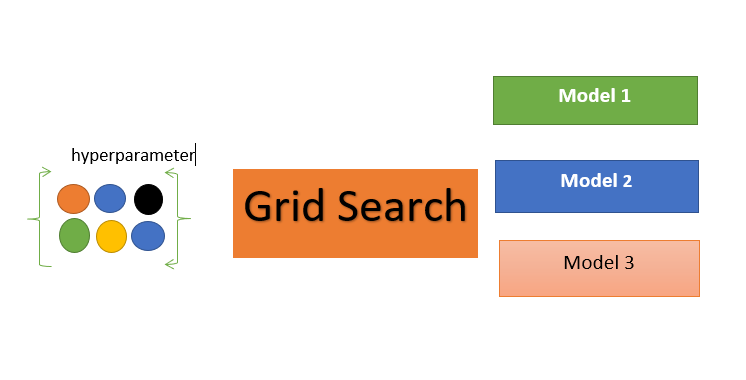Let the tiny circles represent different hyperparameters. We begin with one value for hyperparameters and train the model. We use different hyperparameters to train the model. We tend to continue the method until we have exhausted the various parameter values. Every model produces an error. We pick the hyperparameter that minimizes the error. To pick the hyperparameter, we split our dataset into 3 parts, the training set, validation set, and test set. We tend to train the model for different hyperparameters. We use the error component for each model. We select the hyperparameter that minimizes the error or maximizes the score on the validation set. In ending test our model performance using the test data.

Below we are going to implement hyperparameter tuning using the sklearn library called gridsearchcv in Python.

## Step by step implementation in Python:

a. Import necessary libraries:

Here we have imported various modules like datasets, decision tree classifiers, Standardscaler, and GridSearchCV from different libraries.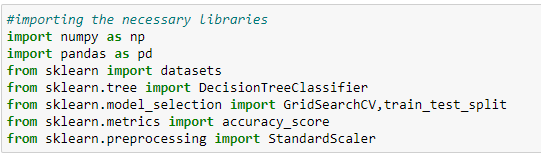We** are looking at **the first five rows of our dataset. In this case, we are using a titanic dataset from Kaggle: There are two files available on Kaggle which train and test CSV.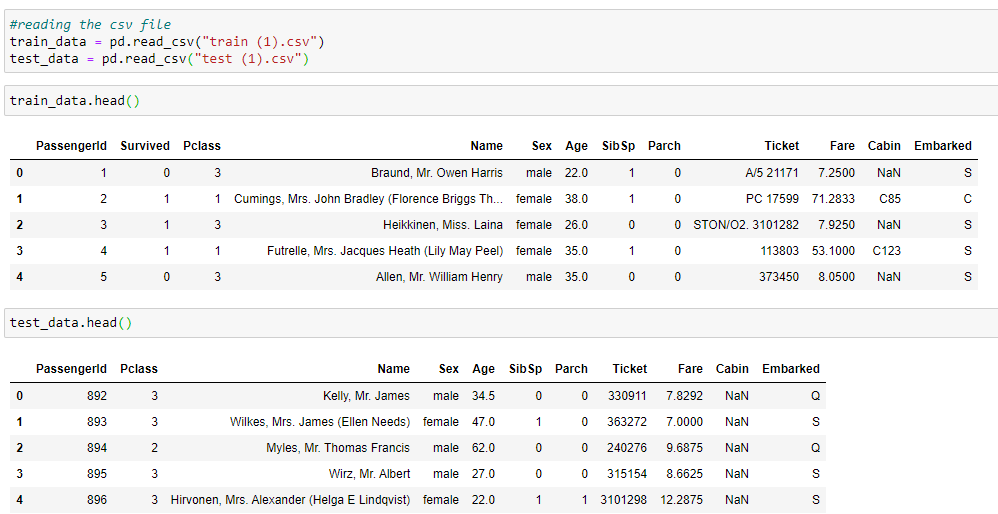c. Removing the unwanted columns.

We have to select specific features that affect the values of the outcome. we can unwanted columns from the train and test dataset.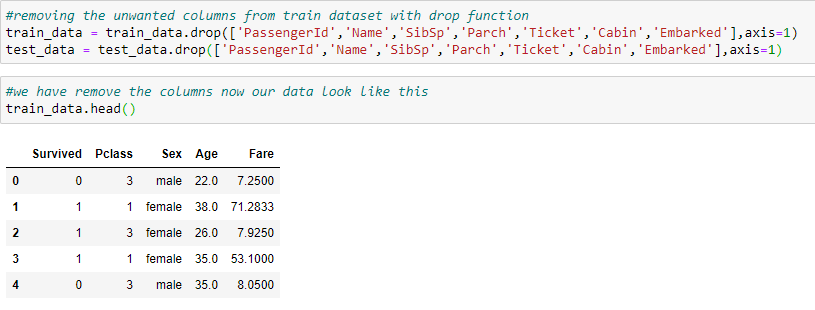d. Exploring the categorical columns.

exploring the categorical columns by replacing the 0s for males and 1s for females. In the decision tree classification problem, we drop the labeled output data from the main dataset and save it as x_train. It is helpful to Label Encode the non-numeric data in columns.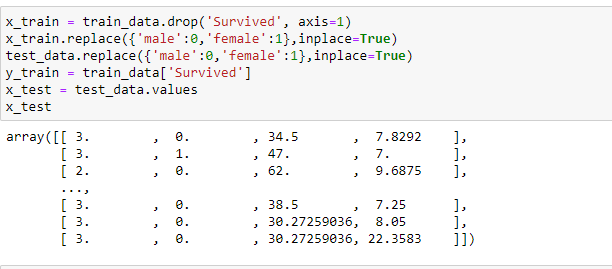e. Removing Null Values.

Sometimes our data contains null values. we have removed the null values before building the classifier model.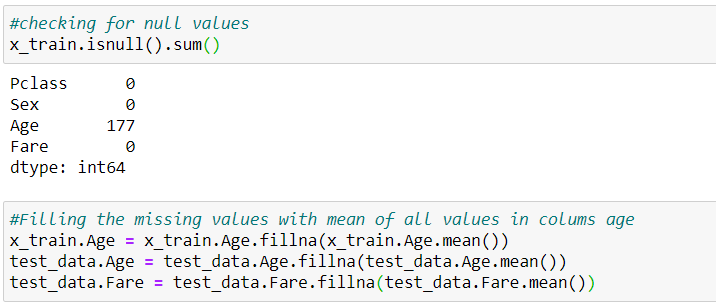f. Training the model.

Here is how we can train our model and do predictions of the test data.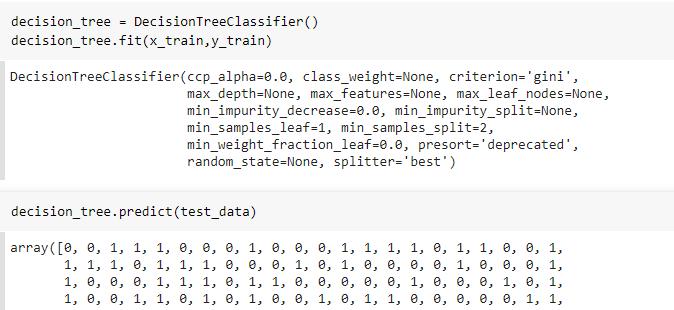g. parameter for gridsearchcv

The value of your Grid Search parameter could be a list that contains a Python dictionary. The key is the name of the parameter. The value of the dictionary is the different values of the parameter. This will make a table that can be viewed as various parameter values. We also have an object or model of the decision tree classifier. The Grid Search is using various kinds of classification performance metrics on the scoring methods. In this case, classification error and number of folds, the model or object, and the parameter values. Some of the outputs include the different scores for different parameter values. In this case, classification error along with parameter values that have the best score.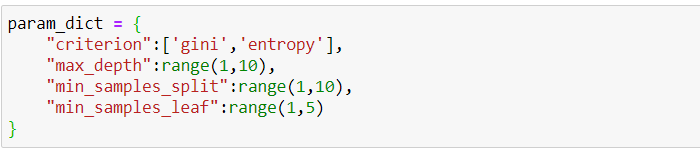h. finding best hyperparameter using gridsearchcv

First, we import the libraries that we need, including GridSearchCV, the dictionary of parameter values. We create a decision tree object or model. We then create a GridSearchCV object. The inputs are the decision tree object, the parameter values, and the number of folds. We will use classification performance metrics. This is the default scoring method. We fit the object. We can find the best values for the parameters using the attribute best estimator. advantages of Grid Search is how quickly we can test multiple parameters.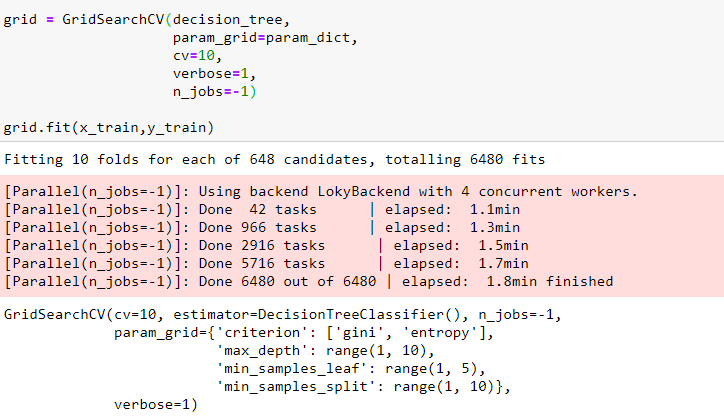In this above code, the decision is an estimator implemented using sklearn. The parameter cv is the cross-validation method if this parameter is set to be None, to use the default 5-fold cross-validation. The parameter verbose controls the verbosity and the parameter n_jobs is the number of jobs to run in parallel. none means 1 unless in a joblib.parallel_backend context. -1 means using all processors.

i. finding the best hyperparameter.

We fit the object. We can find the best values for the parameters using the attribute best estimator.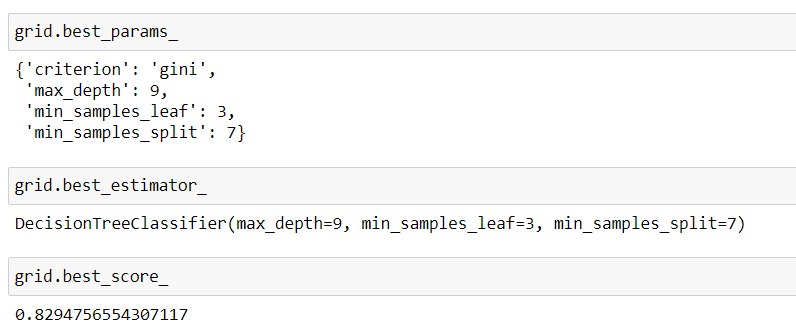References:

Support Vector Machine — Explained

Ensemble Techniques— Bagging (Bootstrap aggregating)

Types of Regression Algorithms

Decision Tree Algorithm In Machine Learning

Naive Bayes Classifier — Explain Intuitively